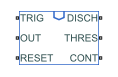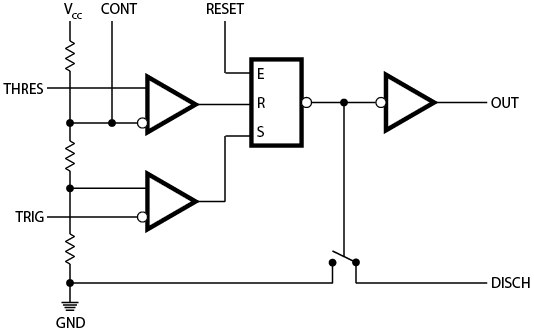# Timer

Behavioral model of a timer integrated circuit

•Libraries:
Simscape / Electrical / Integrated Circuits

## Description

The Timer block is a behavioral model of a timer integrated circuit such as the NE555.

The following figure shows the implementation structure.The Potential divider component resistance parameter sets the values of the three resistors creating the potential divider. The two comparator inputs have infinite input resistance and zero input capacitance. The S-R Latch block provides the functionality of the set-reset latch. It includes an output capacitor and a resistor with values set to match the Propagation delay parameter value. The block models the output stage inverter using a CMOS NOT block. You define the output resistance, low-level output voltage, and high-level output voltage for the CMOS gate in the Timer block dialog box. The discharge switch approximates the NPN bipolar transistor on a real timer as a switch with defined switch on-resistance and off-resistance values.

## Assumptions and Limitations

• The behavior is abstracted. Results are not as accurate as a transistor-level model.

• Delay in response to changing inputs depends solely upon the RC time constant of the resistor-capacitor network at the output of the latch. In practice, the delay has a more complex dependency on the device structure. Set this value based on the output-pulse rise and fall times.

• The drop in output voltage is a linear function of output current. In practice, the relationship is that of a bipolar transistor push-pull pair.

• The controlled switch arrangement used by the block is an approximation of an open-collector arrangement.

• The power supply connects internally within the component, and the block assumes that the GND pin is grounded.

## Ports

### Conserving

expand all

Electrical conserving port associated to the timer threshold pin

Electrical conserving port associated to the timer trigger pin

Electrical conserving port associated to the timer control pin

Electrical conserving port associated to the timer reset pin

Electrical conserving port associated to the timer output pin

Electrical conserving port associated to the timer discharge pin

## Parameters

expand all

### Supply

The voltage value ${V}_{cc}$ that the block applies internally to the timer component.

### Outputs

The output voltage when the timer output is low and no output current is drawn.

The output voltage ${V}_{OH}$ when the timer output is high and no current is drawn.

The ratio of output voltage drop to output current. Set this parameter to $\left({V}_{OH}-{V}_{OH}{}_{1}\right)/{I}_{OH}{}_{1}$, where ${V}_{OH}{}_{1}$ is the reduced output high voltage when the output current is ${I}_{OH}{}_{1}$.

Set this value to the input-pulse or output-pulse rise time.

### Discharge

A representative value is the discharge pin saturation voltage divided by the corresponding current.

A representative value is the discharge pin leakage current divided by the corresponding pin voltage.

### Potential Divider

A typical value for a 555-type timer is 5 kΩ. You can measure it directly across the positive supply and control pins when the chip does not connect to a circuit.

## Version History

Introduced in R2009b